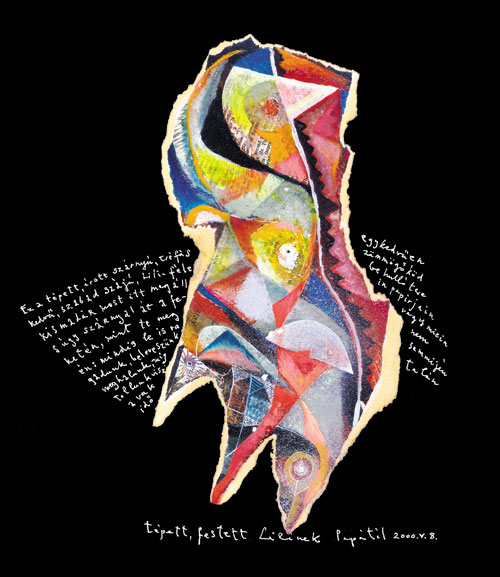TÃ©pett, Ã­rott: Lilinek

E Z Â A Â T Ã‰ P E T T,

Ã R O T T Â S Z Ã R N Y Ãš,

T R Ã‰ F Ã S Â K E D V Å°, Â S Z A B A D Â S Z Ã J Ãš,

L I L I - F Ã‰ L E Â K I S M A D Ã R

M O S T Â I T T Â M E G Ã L L,

S Â Â  Ãš G Y Â  Â S Â  Z Â  Ã Â  R Â  N Â  Y Â  A Â  L

Ã Â  T Â  Â  A Â  Â F Â  E Â  K Â  E Â  T Â  Ã‰ Â  N

M I N T Â T E Â M E G Â Ã‰ N:

M I N D I G Â L E Â I S Â R A G A D U N K,

B E L E V E S Z Ãœ N K, Â M E G H A L U N K,

M Ã G Â TO L L U N K Â V I S Z Â A Â V A K Â I D Å

E G Y H A N G Ãš A N Â Z Ãœ M M Ã– G Å,

K Ã– D B E Â H U L L Ã“ Â T E R E I N,

P A P Ã R J A I N

VA G Y Â M I E I N â€“

N E M

S E M M I J Ã‰ N Â T A L Ã N